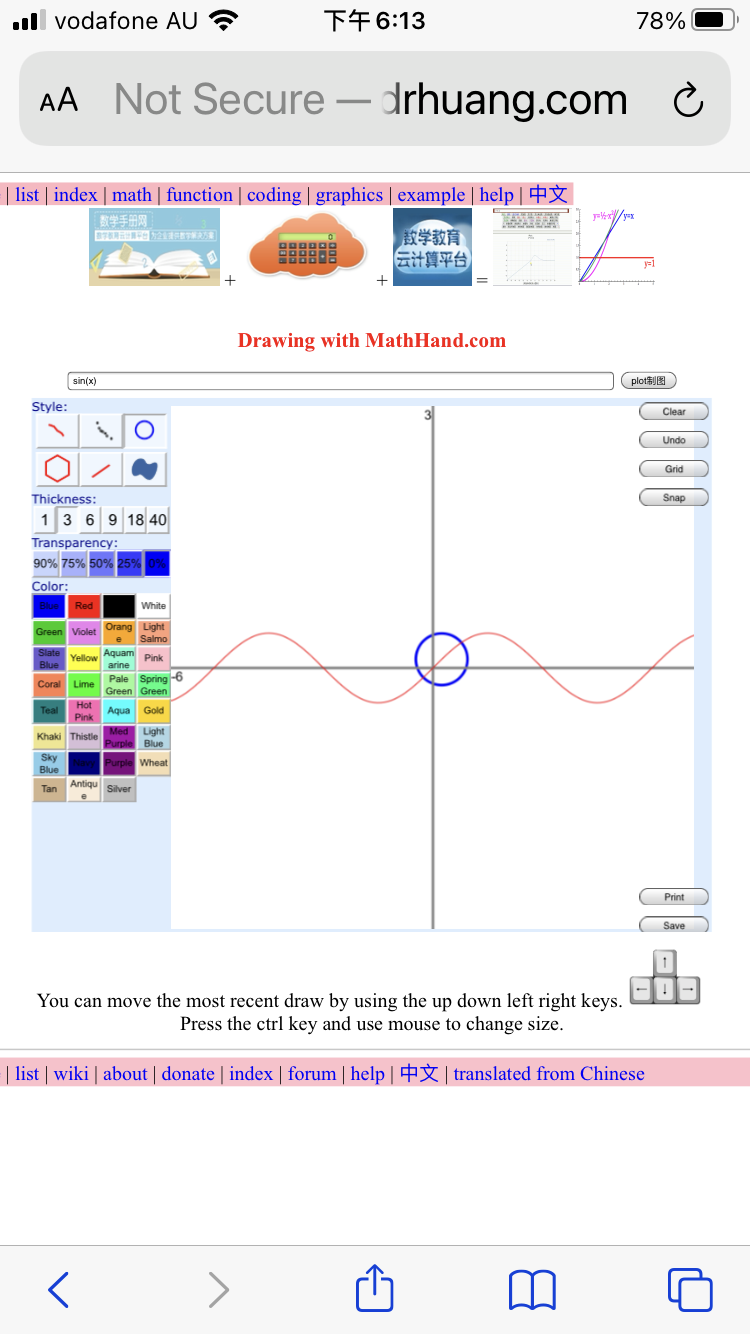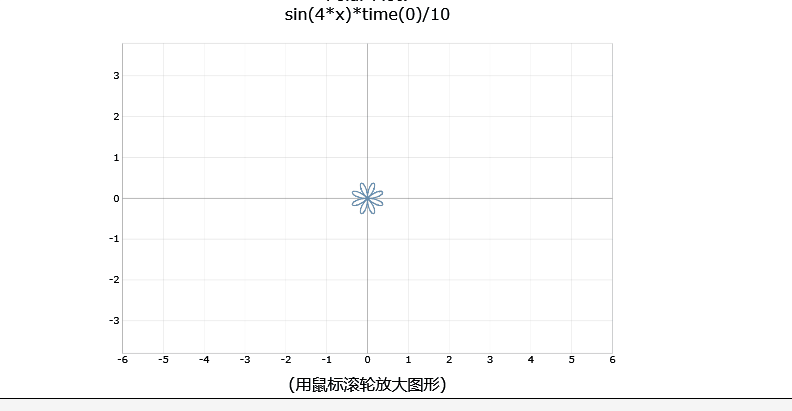﻿ math animation online software ﻿ math handbook calculator - Fractional Calculus Computer Algebra System software+++=# Graphics with online software MathHand.com 制图法 1

## Classification by plotting function 按制图函数分类

### 2D graph

#### plot 制图

The plot( ) plots animation by adding the timer(x) into your formula.
1. sun rise 日升函数 integral(semicircle(timer(3)))
/index/sunrise
2. change the x value to minus, sunrise(-x) become sun down 日落函数 integral(semicircle(timer(-3)))
3. sin wave moving to right when x>0 向右正弦波 sin(x-timer(30))
4. sin wave moving to left when x<0 向左正弦波 sin(x-timer(-30))
5. sine runs after cosine as above two functions are combined 正弦波追余弦波
sin(x-timer(30))+1 and sin(x+timer(30))-1
6. blue peak grow slow and red peak grow fast 篮山峰长高慢 红山峰长高快
gauss(x+2)*timer(5) and gauss(x-2)*timer(10)
7. clock 时钟 timer(-x) and sqrt((9)-x*x)
8. tangent rides on sine wave 切线骑正弦波
sin(x+timer(30)) and cos(timer(30))*x+sin(timer(30))
9. define loving function, call it. 爱你函数
abs(x)^(2/3)+0.9*sqrt(3.3-x^2)*sin(pi*x*timer(30))
10. define a function of damped vibration wave, call it. 阻尼振动波
vibration(t_):=sin(10*x-timer(t))*exp(-0.1*x); vibration(30)

#### polar plot 极坐标图

The polarplot( ) plots animation by adding the timer(x) into your formula.
11. water wave 水波函数 polarplot( timer(6) )
12. Spiral of Archimedes 阿基米德螺线 polarplot( timer(x) )
13. heart become large 心大函数 polarplot( (1-sin(x))*timer(3) )
14. heart become small 心小函数 polarplot( (1-sin(x))*timer(-3) )
15. heart moving 心动函数 polarplot( 1-sin(x-timer(30)) )
16. flower spin 花旋转函数 polarplot( sin(2*x-timer(6)) )
17. flower spin 花旋转函数 polarplot( sin(3*x-timer(6)) )
18. flower spin 花旋转函数 polarplot( sin(4*x-timer(6)) )
19. flowering 开花函数 polarplot( sin(2*x)*timer(6) )
20. flowering 开花函数 polarplot( sin(3*x)*timer(6) )
21. flowering 开花函数 polarplot( sin(4*x)*timer(6) )
22. flowering 开花函数 polarplot( cos(2*x)*timer(6) )
23. flowering 开花函数 polarplot( cos(3*x)*timer(6) )
24. flowering 开花函数 polarplot( cos(4*x)*timer(6) )
25. heartbeat video 心动录像 heart.mp4
26. flowring video 开花录像 flowering.mp4#### plot2D 制图2D

The plot2D( ) plots animation on 4 ways:
1. click the auto-Axis checkbox.
4. On a device with a keyboard, try selecting one of the sliders and holding down a cursor key to see the patterns evolve continuously.

27. plot2D( )
28. plot2D(0.1*cos(x)*x&polar=on&animate=on 贝壳 shell 0.1*cos(x)*x
29. plot2D(x-t&t=10&polar=on&animate=on 顺时针旋转阿基米德螺线 Spiral of Archimedes x-t
30. plot2D(x+t&t=10&polar=on&animate=on 逆时针旋转阿基米德螺线 Spiral of Archimedes x+t
31. plot2D(cos(x-t)+%2b1&autoT=on 向右正弦波 cos(x-t)+1
32. plot2D(cos(x+%2bt)+%2b1&autoT=on 向左正弦波 cos(x+t)+1
33. Cornu Spiral the double Fresnel cosine and sine integrals fresnelC(x),fresnelS(x).
34. Cornu Spiral the Fresnel cosine and sine integrals fresnelC(x),fresnelS(x).
35. plot2D(fresnelC(x)) right-side spring 向右弹簧.
36. plot2D(-fresnelC(x)) left-side spring 向左弹簧.
37. plot2D(S(x),sin(x)-x) down-side spring 向下弹簧.
38. plot2D(S(x),sin(x)+x) up-side spring 向上弹簧.
39. plot2D(S(x),sin(x)-x) double spring 双弹簧.
40. sin(x-timer(9)) 向右正弦波
41. sin(x-timer(-9)) 向左正弦波
42. coloring butterfly 变色蝴蝶
43. coloring square 变色花环
44. Weierstrass Function
45. Unwrapping Circle
46. choreographies
47. x^2-y^2-t
48. color maps

#### Webpage editor

49. solar 太阳系行星运行
50. solar 2D 太阳系行星运行2D
51. taiji 太极图
52. taichi 八卦太极图
53. clock 时钟
54. html clock html 时钟
55. ccs clock ccs 时钟
56. heart beat 心跳
57. I love u 我爱你
58. percent 进度百分比
59. new year 新年
60. driving dog 会开车的狗
61. sea at night 夜里海水
62. fish jumping 鱼跳水
63. firework 烟花
64. rose 玫瑰花
65. calendar 日历
66. Chinese calendar 农历日历
67. mathjs is JavaScript libary
68. react is JavaScript libary

#### Interactive 互动

69. water 可以升降的水
70. sea water 可以升降的海水
71. solar eclipse 日食
72. broke net 打破网
73. love tree 爱心树
74. check code 验证码
75. simple calculator 简单计算器
76. sci calculator 科学计算器
77. color selector 颜色取色器
78. plot 函数图
79. solar3D 互动太阳系3D
80. galaxy 互动太阳系
81. cube

### 3D graphics 立体图

All 3D graphs are interactive, you can zoom, move, spin, etc.
82. cube
83. sphere
84. cylinder
85. bianchi-pinkall-animation 指环
86. bianchi-pinkall-animation-zoomed 大指环
87. FlatTorus
88. solar 3D 互动太阳系
89. galaxy 互动太阳系
90. Metaballs

91. surface3D

#### function3D 制图3D立体图

The function3D( ) plots a function, parametric function in 3D graph.

Here are some particularly nice examples by plot function function3D( )

92. function3D( )
93. function3D(x*y)
94. function3D(x*x, sin(x)) two surfaces overlap

#### plot3D 制图3D

The plot3D( ) plots 3D graph, which become animation by clicking the spin checkbox.
95. plot3D( )

#### parametric3D 函数图3D

96. parametric3D( sinh(u)*sin(v),sinh(u)*cos(v),10*n*v )
97. Parametric Surfaces 25 parametric equations
98. Parametric Surfaces 100 parametric equations
Please refresh this webpage to show different graphics, click the "Parametric Surfaces" to enlarge, click the "equation" to show 200 equations for graphics.

More examples in Surface 3D

#### spin3D 旋转图3D for 4 graphes in one plot

The spin3D plots spin of a function, implicit function, parametric function, a spacial curve, and its inverse in 3D graph.
1. a surface in space. 2. a curve in space. 3. cross-section. 4. positive value of a curve spins to make stereo.

99. spin3D
100. x*x
101. sin(x)
102. cos(x)
103. sin(x*x)
104. cos(x*x)
105. x^2+y^2=1
106. x^2-y^2-1

#### complex3D 复变函数图3D for 20 graphes in one plot

The complex3D( ) plots a function on the complex plane and space, which become animation by clicking the spin checkbox.

Here are some particularly nice examples by plot function complex3D( ) that show real and imaginary parts of complex function including special functions on complex axes and plane.

107. complex3D( )
More examples in complex function

#### wireframe3D 函数图3D

108. wireframe3D( )
More examples in Surface 3D

#### contour3D 函数图3D

109. contour3D( )
More examples in Surface 3D

#### implicit3D 隐函数图3D

110. implicit3D( )
More examples in Surface 3D

### 4D in the space-time of 4 Dimensions

Have you ever tried to see objects in dimension 4? This is possible! Our space is in 3 dimensions. Adding the dimension of time, we get a space-time of dimension 4.

The objects in this space-time are therefore 3D objects which deform with the time. following this principle, this tool proposes to you the visualization of some of these objects as deforming 3D objects, with the aim of helping you to establish this vision in dimension 4.

The implicit3D( ) can plot a 4-dimensional (x,y,z,t) object in 3D space, which become animation by clicking the spin checkbox. You manually change the t value by slider.

111. x*x-y*y-z*z-t-1
More examples in Quadratic hypersurfaces 4D

## Classification by appliaction 按应用分类

<= back - content - Next =>

## Reference 参阅

• function graph 制图
• graphics 制图法
• example 例题 ﻿